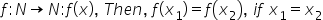Maths-
General
Easy

###Hint:

An injective function (i.e., one-to-one function) is a function for which every element of the range of the function corresponds to exactly one element of the domain. For finding whether the function is injective, we will first assumethen the function is one-one or injective function.

## Book A Free Demo+91